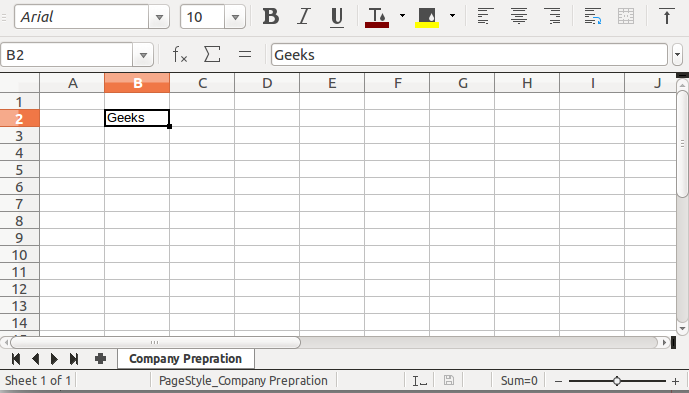Open in App
Not now

# Creating a Cell at specific position in Excel file using Java

• Difficulty Level : Medium
• Last Updated : 30 Jan, 2023

Apache POI is an open-source java library to create and manipulate various file formats based on Microsoft Office. Using POI, one should be able to perform create, modify and display/read operations on the following file formats/ it can be used to create a cell in a Given Excel file at a specific position. Apache POI is an API provided by the Apache foundation.

### Steps to Create a Cell at a Specific position in a given Excel File

1. Create a maven project(Maven is a build automation tool used primarily for Java projects) in eclipse or a Java project with the POI library installed
2. Add the following maven dependency in the pom.xml file
3. Write java code in javaresource folder

Example

## Java

 `// Java Program to Demonstrate Creation Of Cell` `// At Specific Position in Excel File`   `// Importing required classes` `import` `java.io.*;` `import` `org.apache.poi.hssf.usermodel.HSSFWorkbook;` `import` `org.apache.poi.ss.usermodel.Cell;` `import` `org.apache.poi.ss.usermodel.Row;` `import` `org.apache.poi.ss.usermodel.Sheet;` `import` `org.apache.poi.ss.usermodel.Workbook;`   `// Class` `// CreateCellAtSpecificPosition` `public` `class` `GFG {`   `    ``// Main driver method` `    ``public` `static` `void` `main(String[] args)` `        ``throws` `FileNotFoundException, IOException` `    ``{`   `        ``// Creating a workbook instances` `        ``Workbook wb = ``new` `HSSFWorkbook();`   `        ``// Creating output file` `        ``OutputStream os` `            ``= ``new` `FileOutputStream(``"Geeks.xlsx"``);`   `        ``// Creating a sheet using predefined class` `        ``// provided by Apache POI` `        ``Sheet sheet = wb.createSheet(``"Company Preparation"``);`   `        ``// Creating a row at specific position` `        ``// using predefined class provided by Apache POI`   `        ``// Specific row number` `        ``Row row = sheet.createRow(``1``);`   `        ``// Specific cell number` `        ``Cell cell = row.createCell(``1``);`   `        ``// putting value at specific position` `        ``cell.setCellValue(``"Geeks"``);`   `        ``// Finding index value of row and column of given` `        ``// cell` `        ``int` `rowIndex = cell.getRowIndex();` `        ``int` `columnIndex = cell.getColumnIndex();`   `        ``// Writing the content to Workbook` `        ``wb.write(os);`   `        ``// Printing the row and column index of cell created` `        ``System.out.println(``"Given cell is created at "` `                           ``+ ``"("` `+ rowIndex + ``","` `                           ``+ columnIndex + ``")"``);` `    ``}` `}`

Output: On console

`Given cell is created at (1,1)`

Output: Inside file named ‘Geeks.xlsx’My Personal Notes arrow_drop_up
Related Articles